Confidence Intervals for Parameters

There are two methods of computing confidence intervals for the regression parameters. One is based on the profile-likelihood function, and the other is based on the asymptotic normality of the parameter estimators. The latter is not as time-consuming as the former, since it does not involve an iterative scheme; however, it is not thought to be as accurate as the former, especially with small sample size. You use the CLPARM= option to request confidence intervals for the parameters.

Likelihood Ratio-Based Confidence Intervals

The likelihood ratio-based confidence interval is also known as the profile-likelihood confidence interval. The construction of this interval is derived from the asymptoticdistribution of the generalized likelihood ratio test (Venzon and Moolgavkar, 1988). Suppose that the parameter vector is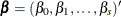and you want to compute a confidence interval for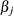. The profile-likelihood function for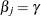is defined as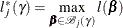where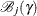is the set of allwith the jth element fixed at, and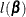is the log-likelihood function for. If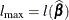is the log likelihood evaluated at the maximum likelihood estimate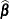, then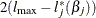has a limiting chi-square distribution with one degree of freedom ifis the true parameter value. Let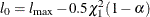, where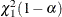is thepercentile of the chi-square distribution with one degree of freedom. A% confidence interval foris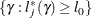The endpoints of the confidence interval are found by solving numerically for values ofthat satisfy equality in the preceding relation. To obtain an iterative algorithm for computing the confidence limits, the log-likelihood function in a neighborhood ofis approximated by the quadratic function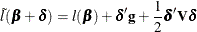where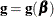is the gradient vector and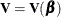is the Hessian matrix. The increment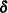for the next iteration is obtained by solving the likelihood equations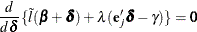whereis the Lagrange multiplier,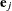is the jth unit vector, andis an unknown constant. The solution is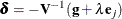By substituting thisinto the equation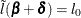, you can estimateas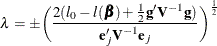The upper confidence limit foris computed by starting at the maximum likelihood estimate ofand iterating with positive values ofuntil convergence is attained. The process is repeated for the lower confidence limit by using negative values of.

Convergence is controlled by the valuespecified with the PLCONV= option in the MODEL statement (the default value ofis 1E–4). Convergence is declared on the current iteration if the following two conditions are satisfied: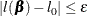and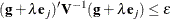Wald Confidence Intervals

Wald confidence intervals are sometimes called the normal confidence intervals. They are based on the asymptotic normality of the parameter estimators. The% Wald confidence interval foris given by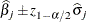where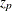is the 100p percentile of the standard normal distribution,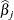is the maximum likelihood estimate of, and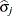is the standard error estimate of.